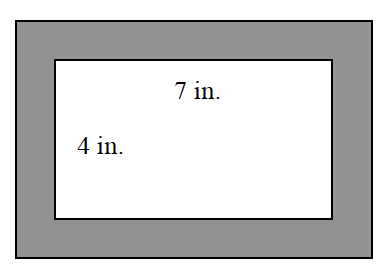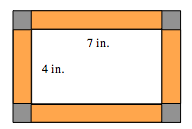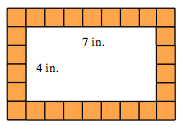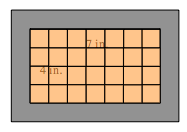### Home > ACC6 > Chapter 4 Unit 4 > Lesson CC1: 4.2.1 > Problem4-48

4-48.

Tina is going to put $1$-inch square tiles on the picture frame shown below.1. If the frame is one tile wide, how many $1$-inch-square tiles will she need?

• To find the number of tiles, it may be useful to find the perimeter of the picture. Remember that the perimeter is the distance around something, so you will know how many tiles fit around the picture. If you use this strategy, do not forget to account for the corner tiles.
The frame is divided so the square corners are shaded gray, and the rectangle pieces between the corners, are shaded orange.

You can also draw one inch square tiles with each side. This way you can count the tiles Tina needs. The 4 rectangles are divided into same size squares as the corners, with 9 squares across the top & bottom, & 6 across the left & right sides, counting the corners in each direction.1. Would more $1$-inch square tiles fit inside the frame or on the frame? Show how you know.

•